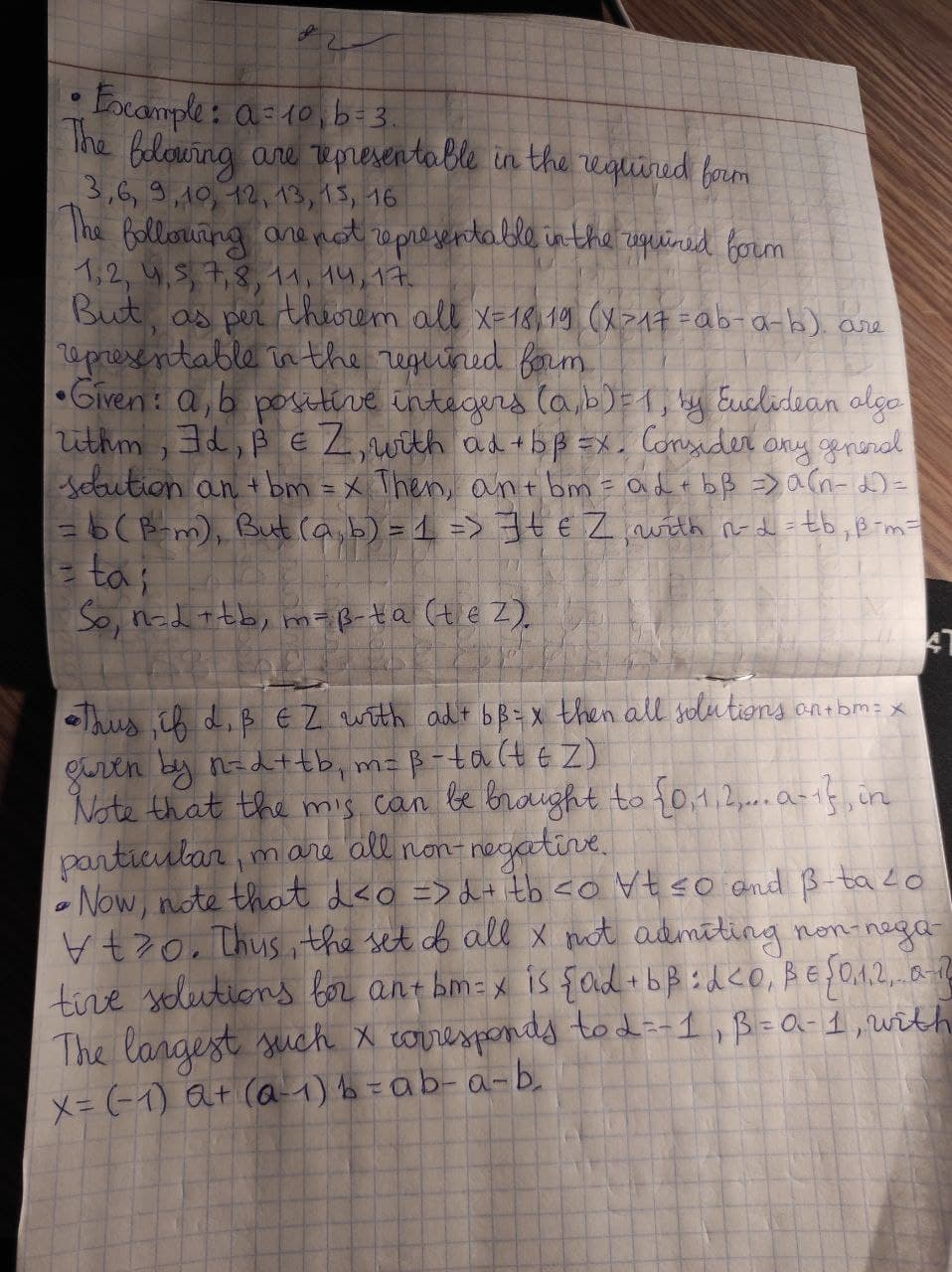# Let a,b be coprime integers. Prove that every integer x>ab-a-b can be written as na+mb where n,m are non negative integers. Prove that ab-a-b cannot be expressed in this form.Joni Kenny 2021-01-05 Answered

Let a,b be coprime integers. Prove that every integer $$x>ab-a-b$$ can be written as $$na+mb$$ where n,m are non negative integers. Prove that $$ab-a-b$$ cannot be expressed in this form.

• Questions are typically answered in as fast as 30 minutes

### Plainmath recommends

• Get a detailed answer even on the hardest topics.
• Ask an expert for a step-by-step guidance to learn to do it yourself.Mayme

We work under the given condtions, a, b positive coprime integers, gcd $$(a,b)=1$$. By Euclidean algorithm, we know that ANY integer x can be represented as $$an+bm$$ . The point is that we require that n and m be non-negative integers. To explain this, consider the example $$a =10,b=3$$. The statement says that every integer $$x > 30-10-3=17$$ can be represented thus. Note that the integer 13 (less than 17) is also of this form , but 17 cannot be represented thus. We need to prove it in the general case.
Example: $$a=10, b=3$$.
The following are representable in the required form 3,6,9,10,12,13,15,16
The following are not representable in the required form 1,2,4,5,7,8,11,14,17.
But, as per theorm. all $$x=18,19 (x>17=ab-a-b)$$ are representable in the required form.
Describing all integral solutions (no conditions) of the equation $$an+bm =x$$. The last line expresses all the solutions (n,m) in terms of a particular solution $$\displaystyle{\left(\alpha,\beta\right)}$$ , we have crucially used that a and b are relatively prime.
Given: a,b positive integers, $$(a,b)=1$$
By Euclidean algorithm, $$\displaystyle\exists\alpha,\beta\in\mathbb{Z}$$, with $$\displaystyle{a}\alpha+{b}\beta={x}{Z}$$
Consider any general solution
$$an+bm=x$$ Then $$\displaystyle{a}{n}+{b}{m}={a}\alpha+{b}\beta{r}{A}{r}{\mathtt{{a}}}{\left({n}-\alpha\right)}={b}{\left(\beta-{m}\right)}$$
But $$\displaystyle{\left({a},{b}\right)}={1}\Rightarrow\exists{t}\in\mathbb{Z}$$, with $$\displaystyle{n}-\alpha={t}{b},\beta-{m}={t}{a}$$,
So, $$\displaystyle{n}=\alpha+{t}{b},{m}=\beta={t}{a}{\left({t}\in\mathbb{Z}\right)}$$
Thus, if $$\displaystyle\alpha,\beta\in\mathbb{Z}$$ with $$\displaystyle{a}\alpha+{b}\beta={x}$$, then all solutions $$an+bm=x$$, given by $$\displaystyle{n}=\alpha+{t}{b},{m}=\beta={t}{a}{\left({t}\in\mathbb{Z}\right)}$$
Note, that the m's can be brought to {0,1,2,...,a-1} In particular, m are all non-negative
We have shown that the largest x for which the representation $$an+bm=x$$ with n, m non-negative is not possible is $$x=ab-a-b$$. In other words, if $$x>ab-a-b$$, then x can be represented as $$an+bm$$ , for some non-negative integers a and b.
Now, note that $$\displaystyle\alpha{<}{0}\Rightarrow\alpha+{t}{b}{<}{0}\forall{t}\le-{\quad\text{and}\quad}\beta-{t}{a}{<}{0}\forall{t}\ge{0}.$$
Thus, the set of all x not admitting non-negative solutions for $$an+bm=x$$ is $$\displaystyle{\left\lbrace{a}\alpha+{b}\beta:\alpha{<}{0},\beta\in{\left\lbrace{0},{1},{2},\ldots,{a}-{1}\right\rbrace}\right.}$$
The largest such x corresponds to $$\displaystyle\alpha=-{1},\beta={a}-{1}$$, with $$\displaystyle{x}={\left(-{1}\right)}{a}+{\left({a}-{1}\right)}{b}={a}{b}-{a}-{b}.$$
We have already proved that x=ab-a-b is not representable in the required form. Here is another proof.
Now, the equation $$an+bm=ab-a-b$$, has a particular solution $$n=-1, m=a-1$$.
So, the general solution is $$n=-1+tb, m=(a-1)-at$$
$$\displaystyle{n}\ge{0}\Rightarrow{t}{b}\ge{1}\Rightarrow{t}\ge{1}$$
But $$\displaystyle{t}\ge{1}\Rightarrow{\left({a}-{1}\right)}-{a}{t}{<}{0}$$
So $$x=ab-a-b$$ cannot be expressed as $$x=an+bm$$,n,m,non-negative integers.